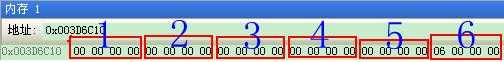﻿ 在C++中用memcpy复制类出错 | Code Bye

# 在C++中用memcpy复制类出错

7年前 (2017-05-08) 2042次浏览
#include<iostream>
using namespace std;
class B
{
public:int num = 0;
B() {};
B(int a)
{
num = a;
}
};
class A
{
int i = 0;
public:B* p = new B;
void create(int n)
{
i++;
B* q = new B[i];
memcpy(q, p, sizeof(p));
delete[] p;
p = new B[i + 1];
memcpy(p, q, sizeof(q));
p[i] = n;
delete[] q;
};
void show(B* b)
{
for (int j = 1; j <= i; j++)
cout << (b + 1)->num << ” “;
}
};
int main()
{
A a;
int i = 0;
cin >> i;
a.create(i);
a.create(3);
a.create(4);
a.create(5);
a.create(6);
a.show(a.p);
return 0;
}

15

memcpy(q, p, sizeof(p));仅仅是拷贝了两个指针值

q = p完全相同的效果

B* q = new B[i + 1];
memcpy(q, b, sizeof(B)*i);
q[i++] = n;
delete [] p;
p = q;

q = p 应该和 memcpy(&q, &p, sizeof(p)) 是一样的。

5

cout<<(b+1)->num<<’’;
b的值是0x003D6C10，该地址的内容如下cout<<(b+j)->num<<’’;

B* p = new B;

B* p = new B(1);

CodeBye 版权所有丨如未注明 , 均为原创丨本网站采用BY-NC-SA协议进行授权 , 转载请注明在C++中用memcpy复制类出错

[1034331897@qq.com]